# Texas Go Math Grade 1 Lesson 13.5 Answer Key Use Related Facts

Refer to our Texas Go Math Grade 1 Answer Key Pdf to score good marks in the exams. Test yourself by practicing the problems from Texas Go Math Grade 1 Lesson 13.5 Answer Key Use Related Facts.

## Texas Go Math Grade 1 Lesson 13.5 Answer Key Use Related Facts

Explore

What number can you add to 8 to get 10? Draw a picture to solve. Write the unknown number.
8 + ________ = 10
Add 2 to number 8 to get 10

FOR THE TEACHER • Read the following problem. Dean has number cards for 8 and 10. What number card would Dean need to add to 8 to get 10?
Dean has number cards for 8 and 10.
The number card 2 is needed to Dean to add to 8 to get 10.

Math Talk
Mathematical Processes

Describe how to solve this problem cubes.

Model and Draw

You can use an addition fact to find a related subtraction fact.
Find 10 – 3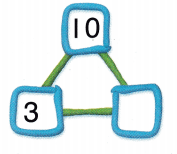3 + _______ = 10
10 – 3 = _________
I know that 3 + 7 = 10, So 10 – 3 = 7.
The unknown number is 7.

Share and Show

Write the unknown numbers.

Question 1.
Find 14 – 8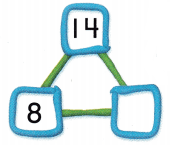8 + ________ = 14
14 – 8 = _________
The unknown number is 6.

Question 2.
Find 17 – 8.8 + ___________ = 17
17 – 8 = _________

The know number is 9.

Question 3.
Find 11 – 6.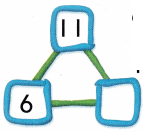6 + _________ = 11
11 – 6 = __________
The unknown number is 5.

Question 4.
Find 15 – 9.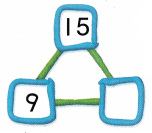9 + __________ = 15
15 – 9 = __________
The unknown number is 6.

Problem Solving

Write the unknown numbers.

Question 5.
Find 20 – 10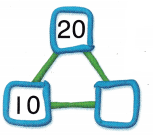10 + _________ = 20
20 – 10 = __________
The unknown number is 10.

Question 6.
Find 13 – 4.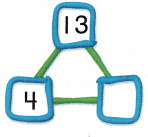4 + _________ = 13
13 – 4 = _________
The unknown number is 9.

Question 7.
H.O.T.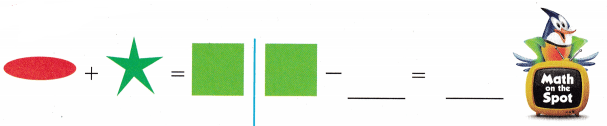H.O.T. Multi-Step Write an addition sentence to help you find the difference. Then write the related subtraction sentence to solve.

Question 8.
Find 11 – 5.
________ + ________ = _________
________ – _________ = _________
5 + 6 = 11
11 – 5 = 6

Question 9.
Find 13 – 6.
_________ = ________ + _________
_________ = ________ + _________

13 = 7 + 6
7 = 13 – 6

Question 10.
15 baby bears brush their teeth before going to bed. 8 baby bears still need to brush their teeth. How many baby bears have already brushed their teeth?
15 – 8 = _________
8 + _________ = 15
(A) 7
(B) 6
(C) 1
Given that,
Baby bears brush their teeth before going to bed = 15.
Baby bears still need to brush their teeth = 8
Baby bears have already brushed their teeth = 15 – 8 = 7
Option A is correct.

Question 11.
Analyze Hanna has II baseball cards. She gives 5 away. She wants to know how many are left. Which number sentence will help you find the missing number?
(A) 11 + 5 = 16
(B) 5 + 6 = 11
(C) 6 – 5 = 1
Given that
The total number of baseballs near Hanna = 11
The total number of baseballs away from Hanna = 5
Number of baseballs left = 11 – 5 =6
5 + 6 = 11number sentence will help to find the missing number

Question 12.
John has 10 books. He reads some books. He has 6 books left to read. Which shows the unknown number of books John has read?
(A) 10 – _________ = 6
(B) 10 – _________ = 2
(C) 4 + _________ = 8
John has books to read = 10
He has books left to read = 6
10 – 6 = 4
4 number of books are read by John.
10 – 4 = 6 expression is correct.

Question 13.
TEXAS Test Prep Which addition fact helps you solve 17 – 8?
(A) 8 + 7 = 15
(B) 9 + 8 = 17
(C) 9 + 9 = 18
9 + 8 = 17 equation helps to solve the 17 – 8
9 + 8 = 17
9 = 17 – 8

TAKE HOME ACTIVITY • Give your child 5 small objects, such as paper clips. Then ask your child how many more objects he or she would need to have 12.
Your child has small objects such as paper clips = 5.
How many objects does he need to get 12 objects are 12 – 5 = 7
7 objects are needed to have 12 objects.

### Texas Go Math Grade 1 Lesson 13.5 Homework and Practice Answer Key

Write the unknown numbers.

Question 1.
Find 16 – 7.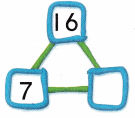7 + ________ = 16
16 – 7 = ___________
The unknown number is 9.

Question 2.
Find 12 – 55 + __________ = 12
12 – 5 = ___________
The unknown number is 7.

Question 3.
Find 14 – 66 + ________ = 14
14 – 6 = __________
The unknown number is 8.
Question 4.
Find 13 – 8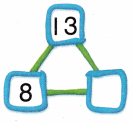8 + __________ = 13
13 – 8 = ___________
The unknown number is 5.

Problem Solving

Multi-Step Write an addition sentence to help you find the difference. Then write the related subtraction sentence to solve.

Question 5.
Find 17 – 9
________ + ________ = __________
________ – ________ = __________
9 + 8 = 17
17 – 9 = 8

Question 6.
Find 15 – 6
__________ = __________ + _________
__________ = __________ – _________
15 = 9 + 6
9 = 15 – 6

Lesson Check

Question 7.
Tina washes 16 dishes after dinner. She still needs to wash 9 of the dishes. How many dishes has Tina already washed?
16 – 9 = _____________
9 + __________ = 16
(A) 7
(B) 9
(C) 8
Tina washes 16 dishes after dinner.
she still has 9 dishes to wash.
Tina already washed = 16 – 9 = 7
A is the correct option

Question 8.
Nate has 12 model planes. He paints some planes. He has 5 pIanes left to paint. Which shows how many planes Nate has left to paint?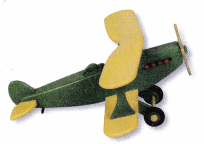(A) 12 – ________ = 8
(B) 5 + ________ = 7
(C) 12 – ________ = 5
Nate has 12 model planes.
She paints some plans and 5 planes are left to paint.
Planes paint to left = 12 – 5 = 7

Question 9.
Which addition fact helps you solve 15 – 7?
(A) 7 + 9 = 16
(B) 7 + 8 = 15
(C) 6 + 9 = 15

7 + 8 = 15 helps to solve the 15 – 7.
From the above equation
15 – 7 = 8.

Question 10.
Mark wants to find 13 – 5. Which shows the related subtraction sentence?
(A) 13 – 8 = 5
(B) 13 – 9 = 4
(C) 13 – 6 = 7